CATEGORIES:

# Matrix. Operations with matrices. The inverse matrix. Determinants and their properties. Systems of linear equations

Theoretical questions:

1. The concept of matrix;

2. Matrix operations: addition of matrices, multiplication of a matrix by a number, matrix multiplication;

3. Calculating determinants of 2×2 and 3×3 matrices;

4. Solving systems of linear equations matrix method;

5. Solving systems of linear equations on Cramer's rule;

6. Investigation compatible system;

7. Solving systems of linear equations Gauss's method.

Classroom assignments:

1. Matrixesandare given. Find 3А+2В.

2. Find matrix Х, if: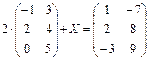3. Calculate: а)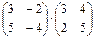;

б) АВ, if: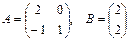.

4. Calculate АВ and ВА, if:5. Calculate D= ABC-3E, if: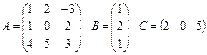6. Find the values ​​of matrix polynomials:

а)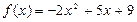, если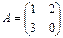б)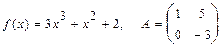в)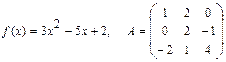7. Calculate determinants:8. Prove that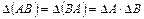, if9. Solve systems of linear equations matrix method by matrix method, by Cramer’s formula, investigate systems on compatibility, and solve them by Gauss's method:

a.b.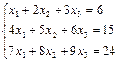c.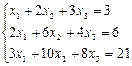10. Investigate systems on compatibility and, in case of compatibility, solve them by Gauss's method:

a.b.c.Homework.

Theoretical material: The simplest problem of analytic geometry. Equations of a straight line on a plane. Vectors.

Solve problems:

1.Calculate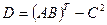, if:2.Find АВС, if:3.Prove, that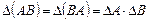, if:4.Solve systems of linear equations matrix method by matrix method and by Cramer’s formula:

a.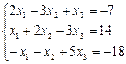b.5. Investigate systems on compatibility and, in case of compatibility, solve them by Gauss's method:Date: 2015-01-02; view: 1031

 <== previous page | next page ==> Trigonometric form of a complex number, de Moivre's formula | PRACTICAL CLASS № 3-5.
doclecture.net - lectures - 2014-2022 year. Copyright infringement or personal data (0.012 sec.)